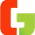## Sunday, September 27, 2020

I keep on playing with my daughter giving her some Mathematical brain teasers. In these brain teasers, one has to find the logic to find the correct answers. Many of these Brain Twisters requires out of box thinking. However, in this post, I will be posting some of the easy Riddles so that kids and teens enjoying solving these puzzles. If you are looking for tough brain teasers then you can check out the Fun Brain Teasers to Challenge your Mind and Out of Box Thinking Fun Brain Teasers.
To avoid the spoilers, answers of each of these mathematical puzzles are not given in this page and links to answers to these puzzles is given at the end of each puzzle. Scroll down till the end of the linked page to check the answers of these brain puzzles.

1. IF
AT=4
CAT=6
CROW=8
BRAIN=10 Then
TWISTER =?

Many people fail this simple logic test. This Brain Teaser looks easy, but the answer to this tricky riddle may surprise you.
2. IF
1= 11
2= 22
3= 33
4= 44
5= 55
6= 66 THEN
11=??

This one is created by my student Shubh and my daughter Evanthe who is 7 years old and 10 years old respectively. So hoping that most of you will be able to do it quickly
3. IF
22x3 = 12
34x2 = 24
15x10 = 50
35x15 = 225 THEN
10x40 = ?

4. One has to find the missing number in the series
3 5 9 15 23 33 ? 59

Don't forget to check out more Find the Next Number in Sequence Puzzles.
5. Again very simple Maths Brain Teaser
IF
2x3x1 = 7
4x5x3 = 23
6x2x5 = 17
3x8x6 = 30
THEN
7x4x7 =?

6. Another easy to do Mathematical Brain Twister which kids will love to do
IF
23 = 44 = 58
13 = 23 = 45
45 = 11 = 92
THEN
34 = 72 = ??

7. Let us continue with the simple riddles.
IF
B+3 = 6
D+2 = 8
E+1 = 5
C+4 = 12
THEN
A+5 =?

8. This one is Fun Brain Teaser
IF
2 = 2
3 = 6
4 = 12
5 = 20
6 = 30
THEN
8 =?

9. I have been giving these brain questions to kids and they are very quick to solve these one. Following brain teaser, they solved it very quickly. Let us see how much time you take to solve this one?
IF
23 - 35 = 58
56 - 21 = 77
87 - 94 = 1711
THEN
56 - 34 = ??

10. This brain puzzle is for children, as this is a very easy one.
IF
2 x 1 = 23
2 x 3 = 65
4 x 2 = 86
5 x 4 = 209
THEN
6 x 3 = ???

11. Find the next numbers in the series
1 2 2 4 3 6 4 8 5 10 ? ?

12. Hopefully, you are having fun while solving these brain twisters. By the time you solve the previous riddles, you have now start thinking out of the box and now you will be able to solve quickly the remaining mind-twisting question. Let us see if you are able to increase your solving speed?
IF
22 = 40
35 = 82
81 = 97
43 = 71
56 = 111
THEN
89 = ??

13. Till now we are playing with numbers, let us play with alphabets. This Brain Puzzle should be easy for you.
IF
A + C = 4
D + E = 9
B + F = 8
Z + C = 29
THEN
G + A = ???

14. Let us give some twist to your mind. This riddle will be able to make you think for some time.
IF
Work is Fun = 5 6 4
Time is Money = 5 3 7
Time is Fun = 3 5 6
THEN
Work = ??? and
Money = ????

15. After a interesting tough question, lets move to easy fun puzzle
IF
2 x 1 x 3 = 5
4 x 5 x 2 = 14
3 x 3 x 4 = 15
5 x 3 x 2 = 11
THEN
6 x 7 x 1 = ?

If you like these puzzles don't forget to check similar Mathematics Logical Mind Cracking Puzzles.

1.Question no. 13 is wrong please make it correct and reply with right one.

1.You are right. I have corrected this puzzle now. Thanks for pointing this out. Keep solving and posting your comments.

2.what is the answer in number 6?

1.I think it is 8.

3.4.1.You can check the answers to this puzzle question in the Part 1 link as given in this post.

5.What is the answer of Question 6?

1.You can click on the Part 2 Answers link to check out answers of 6th to the 10th puzzle questions.

6.cool brain teasers they are a lot of fun :3

7.Could you help me to solve this one:
1= 2
26 = 3
79 = 4
3 = 5

8.Could you help me to solve this one:
1= 2
26 = 3
79 = 4
3 = 5
? = 6

1.Can you please re-check the first term 1=2 or 1=3?

9.Tell us what number 3 is

A comment doesn't cost a thing. Please drop a comment below to boost the author's morale.